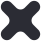Close

# A Level Mathematics

This course is designed so that you will develop your ability to reason logically, extend your range of mathematical skills and use mathematics as an effective means of communication.

You will study Pure Maths which will build upon the topics studied at GCSE. These topics provide the foundation upon which all other modules are based.

Your studies will be supplemented by applications units in Mechanics and Statistics. In Mechanics we look at the forces acting upon objects and the mathematical modelling of these forces. In Statistics we look at the process of data collection and how such data can be analysed.

Mathematics is compatible with many subjects. It is welcomed by Higher Education for admission to degrees in the Sciences, Geography, Economics and Computing, as well as being an essential requirement for Engineering degree courses. It is also popular with employers.

### What will I study?

The exam board is Edexcel (code: 9MA0).

Pure Maths (Year 1)

• Solving equations and inequalities

• Indices and surds

• Curve sketching

• y = mx + c

• Sequences

• Trigonometry

• Exponentials and logs

• Calculus (differentiation and integration)

• Proof

Statistics (Year 1)

• Data presentation, interpretation and use of a large data set

• Statistical distributions

• Probability

• Hypothesis testing

Mechanics (Year 1)

• Vectors

• Kinematics (the motion of an object – speed, acceleration)

• Statics (forces acting on objects which do not move)

• Dynamics (forces acting to move objects)

• Moments

### How will I be assessed?

The course is assessed with 3 exams which are sat at the end of Year 13. All exams are equally weighted and will be 2 hours in length. Two exams will assess Pure Maths. The first Pure Maths unit will be studied in Year 12 and the second one in Year 13. The third exam will be an Applied unit which will contain questions on Mechanics and Statistics. Some of this content will be taught in Year 12 and the rest in Year 13.

## AS Level Further Mathematics

If you have a particular talent and enjoy mathematics then you should consider studying Further Mathematics. You will extend the knowledge you gained through studying the A Level course and encounter new areas of mathematics such as complex numbers, matrices and coordinate systems. The mathematics covered will also be extremely useful to students intending to study Mathematics, Physics or Engineering at degree level. The course builds upon the Pure Mathematics studied in the A Level course and has additional units in Statistics and Decision Maths. Decision Maths looks at the use of algorithms to solve problems. It is an aspect of maths which has developed in line with growth in computer technology. In previous years the completion of the Further Maths course has required a weekly lesson after the college day has finished.

### Course Content and Assessment

The AS Level Further Maths course will have a compulsory content of Pure Maths which will account for 50% of the course. This Pure Maths content will be assessed by two exams at the end of Year 13. The other 50% of the course will contain a further two exams which will assess Statistics and Decision Maths.